Combined Equilibria

Combined Equilibria

A Qualitative View of Combined Equilibria

This section examines how LeChatelier's principle can be applied to systems in which many chemical equilibria exist simultaneously.

Example: Let's consider what happens when solid CuSO4 dissolves in an aqueous NH3 solution. If we start with enough copper sulfate to form a saturated solution, the following solubility product equilibrium will exist in this solution.

CuSO4(s)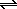Cu2+(aq) + SO42-(aq)

The SO42- ion formed when CuSO4 dissolves in water is a weak Brnsted base that can pick up an H+ ion from water to form the hydrogen sulfate and hydroxide ions.

SO42-(aq) + H2O(l)HSO4-(aq) + OH-(aq)

There are other sources of the OH- ion in this solution. Water, of course, dissociates to some extent to form the OH- ion.

2 H2O(l)H3O+(aq) + OH-(aq)

Ammonia also reacts with water, to some extent, to form the NH4+ and OH- ions.

NH3(aq) + H2O(l)NH4+(aq) + OH-(aq)

The Cu2+ ion released into solution when CuSO4 dissolves reacts with ammonia to form a series of complex ions.

Cu2+(aq) + NH3(aq)Cu(NH3)2+(aq)

Cu(NH3)2+(aq) + NH3(aq)Cu(NH3)22+(aq)

Cu(NH3)22+(aq) + NH3(aq)Cu(NH3)32+(aq)

Cu(NH3)32+(aq) + NH3(aq)Cu(NH3)42+(aq)

If the concentration of the OH- ion is large enough, a second solubility product equilibrium will exist in this solution.

Cu(OH)2(s)Cu2+(aq) + 2 OH-(aq)

The simple process of dissolving copper(II) sulfate in aqueous ammonia therefore can involve nine simultaneous equilibria. In theory, we must consider each of these reactions if we want to predict what will happen under a particular set of experimental conditions. In practice, we can make at least one simplifying assumption. We can assume that the complex ion equilibria in this system can be represented by a single equation in which the Cu2+ ion combines with four NH3 molecules to form the four-coordinate Cu(NH3)42+ ion. We can therefore construct a fairly complete model of what happens in this solution if we take into account the equilibria summarized in the figure below.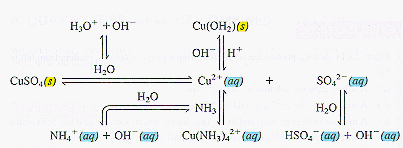Example: Let's use LeChatelier's principle to predict the effect of adding each of the following substances to a saturated solution of CuSO4 in aqueous NH3. We can predict the effect of adding each of these reagents by applying LeChatelier's principle to the above figure.

• CuSO4(s)
Adding excess CuSO4 to a saturated solution has no effect on any of the equilibria shown in the figure because it has no effect on the concentration of either the Cu2+ or SO42- ions.
• HNO3(aq)
Nitric acid is a strong acid that should convert most of the NH3 into NH4+ ions. Anything that removes NH3 from the solution tends to destroy the Cu(NH3)42+ complex ion. Adding nitric acid therefore tends to increase the Cu2+ ion concentration, which should cause CuSO4 to precipitate from solution. We don't expect Cu(OH)2 to precipitate, however, because the concentration of the OH- ion in a strong acid solution is much too small.
• NaOH(aq)
Sodium hydroxide is a strong base. In theory, it should react with the NH4+ ion in this solution to form more NH3. In practice, there isn't very much NH4+ ion in the solution to begin with, so adding NaOH has little effect on most of the equilibria in the solution. The presence of excess OH- ion, however, is likely to precipitate some of the Cu2+ ion in solution as Cu(OH)2.
• Na2SO4(aq)
Sodium sulfate is a source of the SO42- ion. The common-ion effect therefore predicts that some additional CuSO4 will precipitate if Na2SO4 is added to this solution.
• NH3(aq)
Any increase in the NH3 concentration should increase the amount of the Cu2+ ion tied up as Cu(NH3)42+ complex ions. This reduces the concentration of the free Cu2+ ion, which makes the ion product for CuSO4 smaller than the solubility product. As a result, adding NH3 can cause more CuSO4 to dissolve. It is also likely to dissolve any residual Cu(OH)2 that might be present.

Another example of combined equilibria revolves around the aqueous chemistry of the Fe3+ ion. Dilute solutions of this ion prepared by dissolving an iron(III) salt in perchloric acid are essentially colorless. In the presence of the thiocyanate ion, however, a blood-red solution is formed. This solution contains a pair of complex ions.

 Fe3+(aq) + SCN-(aq)Fe(SCN)2+(aq) Kf = 890 Fe(SCN)2+(aq) + SCN-(aq)Fe(SCN)2+(aq) Kf = 2.6

The Fe3+ ion also forms a complex with the citrate ion (Cit3-).

 Fe3+(aq) + Cit3-(aq)Fe(Cit)(aq) Kf = 6.3 x 1011

This neutral coordination complex has a pale yellow color.

Example: The figure below shows a photograph of the following aqueous solutions, reading from left to right. Let's explain the color (or lack thereof) for each of these solutions.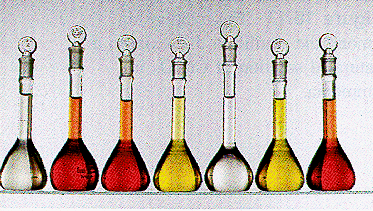(a) A dilute aqueous solution of the Fe3+ ion.
The color of dilute solutions of the Fe3+ ion is so weak they are essentially colorless.

(b) A mixture of the Fe3+(aq) and SCN-(aq) ions.
When the SCN- ion is added to an aqueous solution of the Fe3+ ion, the Fe(SCN)2+ and Fe(SCN)2+ complex ions are formed, and the solution turns a blood-red color.

(c) A mixture of the Fe3+(aq) and SCN-(aq) ions to which a strong acid has been added.
Nothing happens when a strong acid is added to a mixture of the Fe3+ and SCN- ions. This tells us something about the strength of the conjugate acid of the SCN- ionthiocyanic acid, HSCN. If HSCN were a weak acid, adding a strong acid to the solution would tie up the SCN- ion as HSCN. If this happened, the SCN- ion would no longer be free to form a complex with the Fe3+ ion. This would decrease the amount of the Fe(SCN)2+ and Fe(SCN)2+ complex ions in solution, thereby decreasing the intensity of the color of this solution. Since this is not observed, HSCN must be a relatively strong acid.

(d) A mixture of the Fe3+(aq) and Cit3-(aq) ions.
Mixing the Fe3+(aq) and Cit3-(aq) ions forms the Fe(Cit) complex, which has a pale-yellow color.

(e) A mixture of the Fe3+(aq) and Cit3-(aq) ions to which a strong acid has been added.
Because citric acid is a relatively weak acid, the Cit3- ion is a reasonably good base. Adding a strong acid therefore destroys the Fe(Cit) complex by converting the citrate ion into its conjugate acid: citric acid (H3Cit). When this happens, the pale-yellow color disappears.

(f) A mixture of the Fe3+(aq), SCN-(aq), and Cit3-(aq) ions.
The Kf for the Fe(Cit) complex is much larger than Kf for the Fe(SCN)2+ complex ion. Given a choice between SCN- and Cit3- ions, the Fe3+ ions form complexes with Cit3- ions. A solution containing a mixture of these three ions therefore has the characteristic color of the Fe(Cit) complex.

(g) A mixture of the Fe3+(aq), SCN-(aq), and Cit3-(aq) ions to which a strong acid has been added.
When a strong acid is added to a mixture of the Fe3+, Cit3-, and SCN- ions, the Cit3- ions are converted into citric acid (H3Cit). When the Cit3- ions are removed from solution, the only complexing agent left in the solution is the SCN- ion. The solution therefore turns the blood-red color of the Fe(SCN)2+ and Fe(SCN)2+ complex ions.A Quantitative View of Combined Equilibria

We can perform quantitative calculations for solutions of combined equilibria if we keep in mind how these equilibria are coupled.

Example: Let's calculate the solubility of zinc sulfide in a solution buffered at pH 10.00 that is initially 0.10 M in H2S.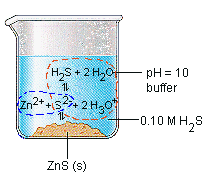We start by building a representation of the equilibria that must be considered in order to do this calculation, such as the one shown above. We then calculate the H3O+ ion concentration in this buffer solution.

[H3O+] = 10-pH = 10-10.00 = 1.0 x 10-10 M

And then consider the effect this pH would have on the dissociation of H2S.

 H2S(aq) + 2 H2O(l)2 H3O+(aq) + S2-(aq) Kc = 1.3 x 10-20

Because it is a weak acid, we can assume that the concentration of H2S at equilibrium is more or less equal to its initial concentration.

[H2S]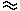0.10 M

We now know the H2S and H3O+ concentrations at equilibrium, which means that we can calculate the concentration of the S2- ion at equilibrium before any ZnS dissolves.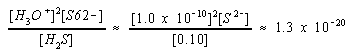When ZnS dissolves, we get more S2- ion. The amount of ZnS that dissolves can be calculated from the following equation, where Cs is the solubility of ZnS.

[Zn2+][S2-] = Ksp

[Cs][Cs + 0.13] = 1.6 x 10-24

If we have to, we can always solve this equation with the quadratic formula. Before we do this, however, we might look for an assumption that make the problem easier to solve. In this case, it seems reasonable to assume that the solubility of ZnS is so small that most of the S2- ion at this pH comes from the dissociation of H2S.

Cs << 0.13 M

When this assumption is made, we get the following approximate equation.

[Cs][0.13]1.6 x 10-24

When we solve this equation, we get a solubility of ZnS that is so small that we have to conclude that the assumption used to generate this equation is valid.

Cs1.2 x 10-23

There is no magic formula that can help us divide problems such as the example above into steps, or decide the order in which steps should be handled. The following general rules, however, might guide us through these problems.

• Identify the equilibria that must be included in the model of the solution.
• Draw a figure that shows how these equilibria are coupled.
• Find the simplest equilibriumthe one for which all of the necessary data are availableand solve this part of the problem.
• Ask: "Where did this get me?" "What can I do with this information?"
• Never lose track of what was accomplished in a previous step.
• Use the results of one step to solve another until all of the equilibria have been solved.

Example: Let's predict whether AgOH will precipitate from a solution buffered at pH 9.00 that is initially 0.010 M AgNO3 and 1.0 M NH4NO3.

 AgOH: Ksp = 2.0 x 10-8 NH3: Kb = 1.8 x 10-5 Ag(NH3)2+: Kf = 1.1 x 107 H2O: Kw = 1.0 x 10-14

We might start by noting that silver nitrate and ammonium nitrate are both soluble salts.

 H2O AgNO3(s)Ag+(aq) + NO3-(aq)
 H2O NH4NO3(s)NH4+(aq) + NO3-(aq)

We then build a representation of the equilibria present in this solution such as the one in the figure below.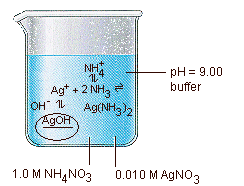We can solve some problems by looking at the initial conditions and working toward the final answer. Others, such as this, are so complex it is useful to look at the goal and then work backwards. The goal in this problem is to decide whether AgOH precipitates from this solution. In order to make this decision, we need two pieces of information: the Ag+ and OH- ion concentrations at equilibrium. We can therefore divide the problem into two parts whose goals consist of determining the values of the [Ag+] and [OH-] terms when all other reactions in this system are at equilibrium.

It seems reasonable to start with the goal that is easiest to achieve: finding the OH- ion concentration. Because we know the pH of this buffer solution, we can calculate the H3O+ ion concentration at equilibrium.

[H3O+] = 10-pH = 10-9.00 = 1.0 x 10-9 M

We can then use this H3O+ ion concentration to calculate the OH- ion concentration in the buffer solution.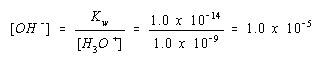We now turn to the second part of the problem, finding the concentration of the Ag+ ion at equilibrium. We know that this ion is in equilibrium with the Ag(NH3)2+ complex ion.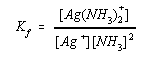If we knew the concentrations of NH3 and the Ag(NH3)2+ complex ion, we could calculate the Ag+ ion concentration at equilibrium. This gives us two new sub-goals: determining the Ag(NH3)2+ and NH3 concentrations.

Determining the Ag(NH3)2+ ion concentration is relatively easy. We know that the initial concentration of the Ag+ ion in this solution is 0.010 M. We also know that this ion forms a strong complex with NH3. We can therefore assume that essentially all of the silver ions in this solution will be present as Ag(NH3)2+ complex ions.

[Ag(NH3)2+]0.010 M

Now all we need is the NH3 concentration. We know that ammonia is in equilibrium with the NH4+ ion.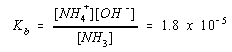And we already know the OH- ion concentration in this solution.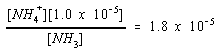Rearranging this equation gives the following result.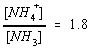The concentration of the NH4+ ion at equilibrium is therefore 1.8 times the concentration of NH3.

[NH4+] = 1.8 [NH3]

We also know that the solution was initially 1.0 M in NH4+ ions. These ions are now present as NH3 molecules, as NH4+ ions, or as part of the Ag(NH3)2+ complex ions.

[NH3] + [NH4+] + 2 [Ag(NH3)2+] = 1.0 M

We can ignore the last term in this equation because the concentration of the Ag(NH3)2+ ion can't be larger than the initial concentration of the Ag+ ion: 0.010 M. We can therefore assume that only a negligibly small fraction of the NH4+ that was added to the solution is present in the Ag(NH3)2+ complex ion.

[NH3] + [NH4+]1.0 M

We now have two equations in two unknowns.

[NH4+] = 1.8 [NH3]

[NH3] + [NH4+] = 1.0 M

Substituting the first of these equations into the second allows us to solve for the NH3 and NH4+ concentrations at equilibrium.

[NH3] = 0.36 M

[NH4+] = 0.64 M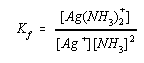and substitute into this equation the approximate values for the concentrations of NH3 and the Ag(NH3)2+ complex ion at equilibrium.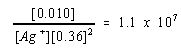We can then solve this equation for the Ag+ ion concentration at equilibrium.

[Ag+]7.0 x 10-9 M

We can now look at the product of the Ag+ and OH- ion concentrations when this system is at equilibrium.

[Ag+][OH-] = [7.0 x 10-9][1.0 x 10-5] = 7.0 x 10-14

The ion product for this solution (7.0 x 10-14) is very much smaller than the solubility product for AgOH (2.0 x 10-8), which means that AgOH won't precipitate from solution.